# Solution assignment 07 Quadratic functions and graphs

### Assignment 7

For which value of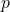does the parabola: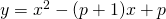have two intersection points with the-axis having a mutual distance of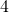?

### Solution

According to the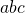-formula, the intersection points with the-axis are: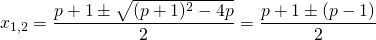So: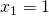or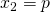The distance between both intersection points isand thus we have to calculatefrom (see also Absolute value |x|):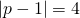so: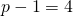of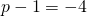so: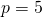or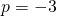0